GFG App
Open AppBrowser
Continue

# Using Request Args for a Variable URL in Flask

This article will teach us how to use Request Arguments in our Flask application. First, we will understand. What are the Request Arguments?

## What are the Request Arguments?

Request Arguments are MultiDict objects with the parsed contents of the query string (the part in the URL after the question mark).

MultiDict:  It is a dictionary-like structure, having key-value pairs, but the ‘same key’ can occur multiple times in the collection.

In Flask, we can use the request.args attribute of the request object to access the URL parameters. These parameters are appended to the end of the URL in the form of key=value, separated by ampersands (&). As we can see in the following image, first_name & last_name are used as the keys, and Raj & Kumar are the values assigned to them.Here is an example of how we can use request.args in a Flask route to access the value of a URL parameter:

Create a new file main.py. Inside it, rewrite the following code.

## Python3

 `from` `flask ``import` `Flask, request`   `app ``=` `Flask(__name__)`   `@app``.route(``'/'``)` `def` `index():` `    ``# Get the value of the 'name' parameter from the URL` `    ``name ``=` `request.args.get(``'name'``)` `    `  `    ``# Greet Hello to name provided in the URL Parameter` `    ``return` `"Hello, {}!"``.``format``(name)` `  `  `app.run()`

Now, Run the main.py

`\$ python main.py `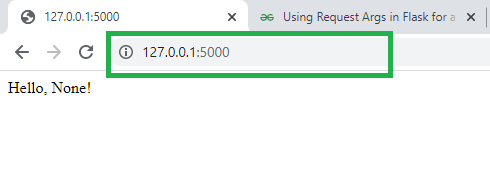In the screenshot, you can see the URL parameter is NOT provided that’s why “Hello, None!” is rendered on the webpage. if we will assign a URL parameter as you can see in the following output.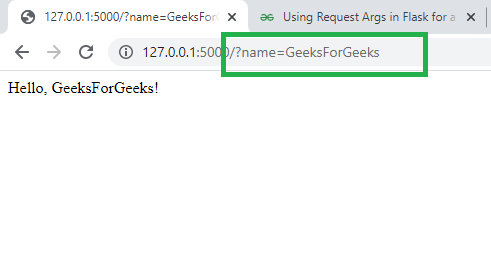In this example, the request arguments key is the “name” and GeeksForGeeks is the value. So, “Hello, GeeksForGeeks!” is printed on the screen. Similarly, if we visit the URL http://127.0.0.1:5000/?name=John, the route will return the string “Hello, John!”. and if we visit the URL http://127.0.0.1:5000/?name=David, the route will return the string “Hello, David!”

Let’s create a mini-application to understand more about the Request Arguments. We will create an Arithmetic Calculator. In which, we will perform different arithmetic operations like Addition, Subtraction, Multiplication, and Division. To do this we will use Request Arguments.

## Create Arithmetic Calculator

To create an arithmetic calculator using request arguments in Flask, we can follow these steps:

• Create a new file calculator.py and define a route for the calculator in Flask app. This route will accept request arguments for the numbers and operation to be performed. For example:

## Python3

 `@app``.route(``'/calculate'``)` `def` `calculate():` `  `  `  ``# Get the first operand using request arguments` `  ``a ``=` `request.args.get(``'a'``) ` `  `  `  ``# Get the Second operand using request arguments` `  ``b ``=` `request.args.get(``'b'``) ` `  `  `  ``# Get the operation to be perform` `  ``operator ``=` `request.args.get(``'operator'``)`

• Now, the parameters we have are in string type. To convert them to integers we can use the int() function. Also, we will ensure that the arguments should not empty.

## Python3

 `# Make sure that the request arguments are not empty` `  ``if` `a ``and` `b ``and` `operator:` `    ``# Convert the request arguments to integers` `    ``a ``=` `int``(a)` `    ``b ``=` `int``(b)`

• Use an if statement to determine which operation to perform based on the value of the operator request argument. We can use the standard arithmetic operators (+, -, *, /) to perform the desired operation.

## Python3

 `# Perform the requested operation` `    ``if` `operator ``=``=` `'add'``:` `      ``result ``=` `a ``+` `b` `    ``elif` `operator ``=``=` `'subtract'``:` `      ``result ``=` `a ``-` `b` `    ``elif` `operator ``=``=` `'multiply'``:` `      ``result ``=` `a ``*` `b` `    ``elif` `operator ``=``=` `'divide'``:` `      ``result ``=` `a ``/` `b`

• Finally, Return the result of the operation to the user. Otherwise, if any argument is missing in the URL then return the “Error: Insufficient arguments”

## Python3

 `  ``return` `f``'{a} {operator} {b} = {result}'` `else``:` `  ``return` `'Error: Insufficient arguments'`

Here is a Complete code:

## Python3

 `from` `flask ``import` `Flask, request`   `app ``=` `Flask(__name__)`   `@app``.route(``'/calculate'``)` `def` `calculate():` `  `  `  ``# Get the first operand using request arguments` `  ``a ``=` `request.args.get(``'a'``) ` `  `  `  ``# Get the Second operand using request arguments` `  ``b ``=` `request.args.get(``'b'``) ` `  `  `  ``# Get the operation to be perform` `  ``operator ``=` `request.args.get(``'operator'``)`   `  ``# Make sure that the request arguments are not empty` `  ``if` `a ``and` `b ``and` `operator:` `    ``# Convert the request arguments to integers` `    ``a ``=` `int``(a)` `    ``b ``=` `int``(b)`   `    ``# Perform the requested operation` `    ``if` `operator ``=``=` `'add'``:` `      ``result ``=` `a ``+` `b` `    ``elif` `operator ``=``=` `'subtract'``:` `      ``result ``=` `a ``-` `b` `    ``elif` `operator ``=``=` `'multiply'``:` `      ``result ``=` `a ``*` `b` `    ``elif` `operator ``=``=` `'divide'``:` `      ``result ``=` `a ``/` `b`   `    ``return` `f``'{a} {operator} {b} = {result}'` `  ``else``:` `    ``return` `'Error: Insufficient arguments'`   `  ``app.run()`

To use this calculator, we can send a GET request to the ‘/calculate’ endpoint with the request arguments ‘a’, ‘b’, and ‘operator’.

For example, to add 2 and 3, we can send a request like this:

`http://localhost:5000/calculate?a=2&b=3&operator=add`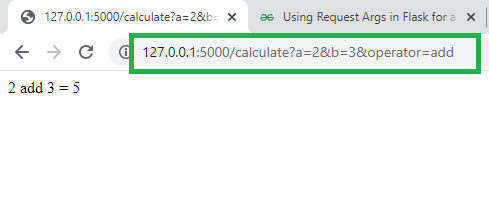To Multiply 4 and 7:

`http://localhost:5000/calculate?a=2&b=3&operator=multiply `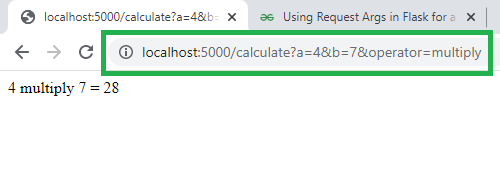Similarly, we can perform Subtraction and Division.

If any of the arguments are missing in the URL, Like

`http://localhost:5000/calculate`

Or

`http://localhost:5000/calculate?a=2&b=3`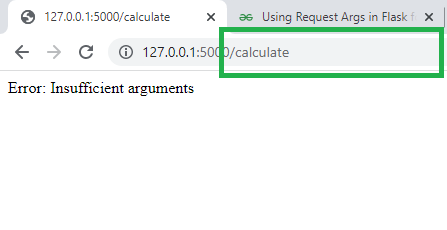My Personal Notes arrow_drop_up